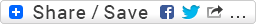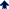Medical Book Would you like to react to this message? Create an account in a few clicks or log in to continue.Medical BookBuy Textbooks | Autoclaves | stethoscopes | Buy Books Online | Buy Medical Textbooks | Textbooks | Equipment | Nutrition | USMLE | MRCP | MRCS | Dental | Sport Medicine | Cardiology | Medical Textbook | Surgery | Pregnancy | Anatomy | Radiation | Pedia |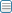Share

# applying risk reward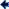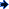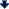AuthorMessage
painofhellPosts : 669
Birthday : 1990-05-18
Join date : 2014-08-16
Age : 30
Job/hobbies : forex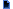Subject: applying risk reward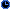Mon Aug 25, 2014 12:42 pm

### Is Risk:Reward the Holy Grail of Strategies?

[th]Risk:Reward = 1:1[/th][th]Risk:Reward = 1:2[/th][th]Risk:Reward = 1:3[/th][th]Risk:Reward = 1:5[/th]
Risk = 2%Risk = 2%Risk = 2%Risk = 2%
Reward = 2%Reward = 4%Reward = 6%Reward = 10%
TotalProfit = 4%Total Profit = 4%TotalProfit = 4%Total Profit = 4%

### For those of you who prefer to view everything in pips instead of percentages here is another table with the exact same information. If you had a plan that allowed you to always limit your stop loss to the exact same amount of pips every time then you could look at it this way. Based on taking 10 trades this is how it would all play out for the same total profit.

[th]Risk:Reward = 1:1[/th][th]Risk:Reward = 1:2[/th][th]Risk:Reward = 1:3[/th][th]Risk:Reward = 1:5[/th]
Risk = 50pipsRisk = 50pipsRisk = 50pipsRisk = 50pips
Reward = 50pipsReward = 100pipsReward = 150pipsReward = 250pips
Total Profit = 100pipsTotal Profit = 100pipsTotal Profit = 100pipsTotal Profit = 100pips

### Earlier on in this lesson you were told that a 70% win rate is very realistic with the approach that has been outlined in these lessons. A higher win rate than that is very possible so let’s assume a lower win rate to be conservative. Based on only ever winning 60% of your trades here is another look at those same risk:reward ratios to compare the outcomes.

[th]Risk:Reward = 1:1[/th][th]Risk:Reward = 1:2[/th][th]Risk:Reward = 1:3[/th][th]Risk:Reward = 1:5[/th]
Risk = 2%Risk = 2%Risk = 2%Risk = 2%
Reward = 2%Reward = 4%Reward = 6%Reward = 10%
Total Profit = 4%Total Profit = 12%Total Profit = 28%Total Profit = 52%

### By always winning 60% of the trades that you attempt you can see the mathematical advantaged that a risk:reward strategy can provide. It’s not complicated, but it is powerful, and it should play a part in your complete trading plan.